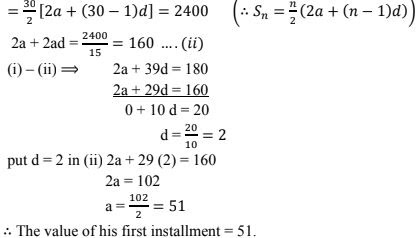# RD Sharma Solutions Chapter 9 Arithmetic Progression Exercise 9.5 Class 10 Maths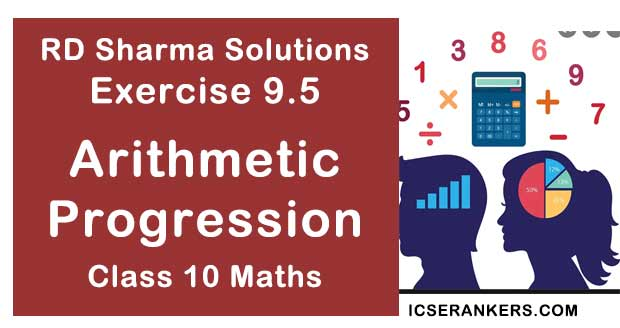Chapter Name RD Sharma Chapter 9 Arithmetic Progression Book Name RD Sharma Mathematics for Class 10 Other Exercises Exercise 9.1Exercise 9.2Exercise 9.3Exercise 9.4MCQ Related Study NCERT Solutions for Class 10 Maths

### Exercise 9.5 Solutions

1. Find the sum of the following arithmetic progressions:
(i) 50, 46, 42, ...... to 10 terms
(ii) 1, 3, 5, 7, ..... to  12 terms
(iii) 3, 9/2, 6, 15/2, ..... to  25 terms
(iv) 41, 36, 31, ..... to 12 terms
(v) (a + b), (a - b), (a - 3b), ..... to 22 terms
(vi ) (x - y)2 ,(x2 + y2 ), (x + y)2 ,......., to n terms
(vii) (x - y)/(x + y), (3x - 2y)/(x + y), (5x - 3y)/(x + y), .... to n terms
(viii) - 26, -24, -22, ..... to 36 terms

Solution

In an A.P
Let,
First term = a,
Common difference = d, and there are n terms. Then, sum of n terms is,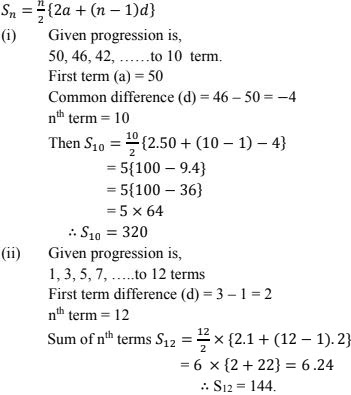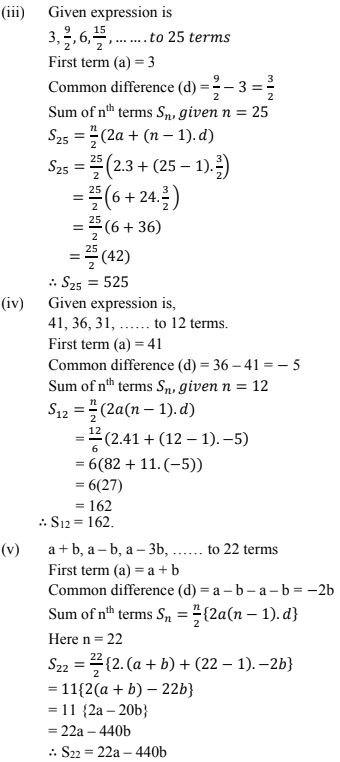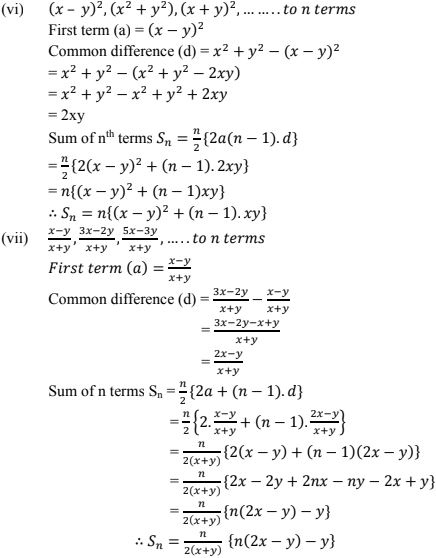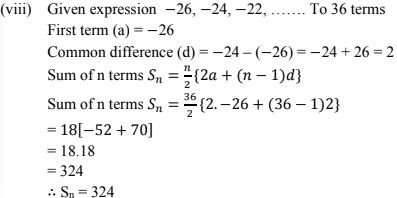2. Find the sum to n term of the A.P. 5, 2, - 1, -4, -7,..........
Solution
Given AP is 5, 2, -1, -4, -7,..........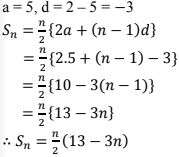3. Find the sum of n terms of an A.P. whose the terms is given by an = 5 – 6n.
Solution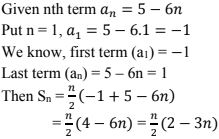4. If the sum of a certain number of terms starting from first term of an A.P. is 25, 22, 19, .... is 116. Find the last term.
Solution
Given AP is  25, 22, 19, .....
First term (a) = 25, d = 22 - 25 = -3.
Given, Sn = n/2(2a + (n - 1)d)
116 = n/2(2×25+(n -1)-3)
⇒ 232 = n(50 - 3(n -1))
⇒ 232 = n(53 - 3n)
⇒ 232 = 53n - 3n2
⇒ 3n2 - 53n + 232 = 0
⇒ (3n - 29)(n - 8) = 0
∴ n = 8
⇒ a8 = 25 + (8 - 1) - 3
25 - 24 = 4
∴ n =8, a8 = 4

5. (i) How many terms of the sequence 18, 16, 14, .......... should be taken so that their
(ii) How many terms are there in the A.P. whose first and fifth terms are -14  and 2 respectively and the sum of the terms is 40?
(iii) How many terms of the A.P. 9, 17, 25, ..... must be taken so that their sum is 636 ?
(iv) How many terms of the A.P. 63, 60, 57, ...... must be taken so that their sum is 693 ?
Solution
(i) Given sequence, 18, 16, 14, .....
a = 18, d = 16- 19 = - 2.
Let, sum of n terms in the sequence is zero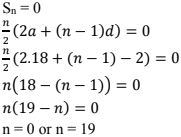(ii) ∵ n = 0 is not possible. Therefore, sum of 19 number in the sequence is zero.
Given, a = - 14, a5 = 2
a + (5 - 1)d = 2
⇒ -14 + 4d = 2
⇒ 4d = 2+ 14 = 16
⇒ d = 16/4 = 4
Sequence is -14, -10, -6, -2, 2, .........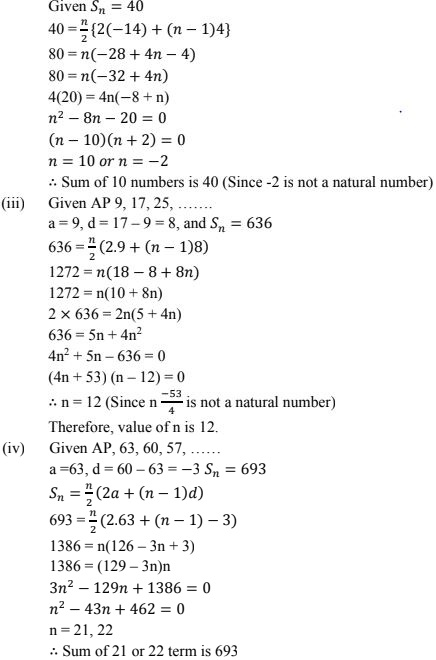6. The first and the last terms of an A.P are 17 and 350 respectively. If the common difference is 9, how many terms are there and what is their sum ?
Solution
Given, a = 17, L = 350, d = 9
L = an  = a + (n - 1)d
350 = 17 + (n - 1)9
⇒ 333 = (n - 1)9
⇒ n - 1 = 333/9 = 37
⇒ n = 38
∴ 38 terms are there
sn  = n/2(a + l)
= 38/2(17 + 350)
= 19.367
∴ sn  = 6973

7. The third term of an A.P is 7 and the seventh term exceeds three times the third term by 2. Find the first term, the common difference and the sum of first 20 terms.
Solution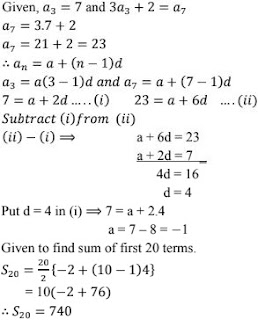8. The first term of an A.P. is 2 and the last term is 50. The sum of all these terms is 442. Find the common difference.
Solution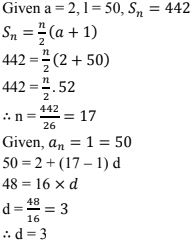9. If  12 th term of an A.P. is  -13 and the sum of the first four terms is 24,  what is the sum of first 10 terms ?
Solution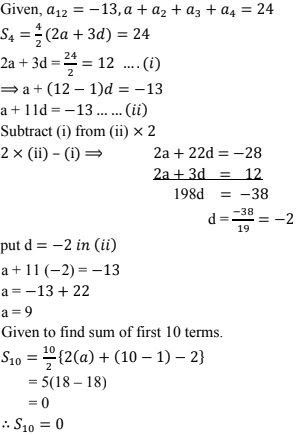10. Find the sum of first 22 terms of an A.P. in which d = 22 and a = 149.
Solution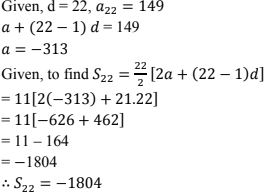11. Find the sum of all natural numbers between 1 and 100, which are divisible by 3.
Solution
The numbers between 1 and  100 which are divisible by 3 are 3, 6, 9, ...  99.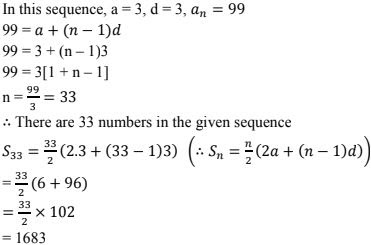∴ Sum of all natural numbers between 1 and 100, which are divisible by 3 is 1683.

12. Find the sum of first n odd natural numbers.
Solution
The sequence is 1, 3, 5, ......, n.
In this first term (a) = 1, common difference d = 3 - 1 = 2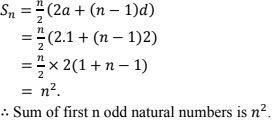13. Find the sum of all odd numbers between (i) 0 and 50  (ii) 100 and 200
Solution
(i) Odd numbers between 0 and 50 are 1, 3, 5, ...., 49
In this a  = 1, d = 2, l = 49 = an
49 = 1 + (n - 1)2  (∴ an  = a + (n - 1)d)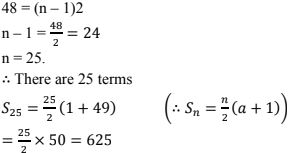∴ Sum of all odd numbers between 0 and 50 is 625.

(ii) Odd numbers between 100 and 200 are 101, 103, ..... 199
In this a = 101, d = 2, l = an  = 199
199 = 101 + (n - 1)2
⇒ n - 1 = 98/2 = 49
⇒ n = 49+ 1 = 50
∴ There are 50 terms.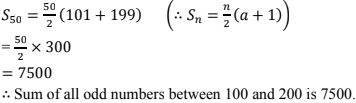14. Show that the sum of all odd integers between 1 and 1000 which are divisible by 3 is 83667.
Solution
Odd integers between 1 and 1000 which are divisible by 3 are 3, 6, 9, 15, ....., 999.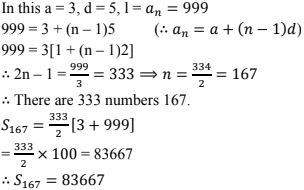∴ Sum of all odd integers between 1 and 4000 which are divisible by 3 is 83667.

15. Find the sum of all integers between 84 and  719, which are muliples of 5.
Solution
The numbers between 84 and 719, which are multiples of 5 are 85, 90, 95, ......, 715.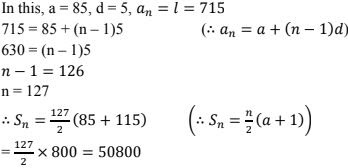∴ Sum of all integers between 84 and 719, which are multiples of 5 is 50800.

16. Find the sum of all integers between 50 and 500, which are divisible by 7.
Solution
Numbers between 50 and 500, which are divisible by  7 are 56, 63, ......, 497.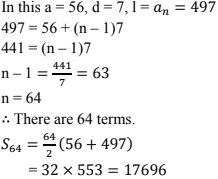∴ Sum of all integers between 50 and 500, which are divisible by 7 is 17696.

17. Find the sum of all even integers between 101, and 999.
Solution
Even integers between 101 and 999 are  102, 104, ....., 998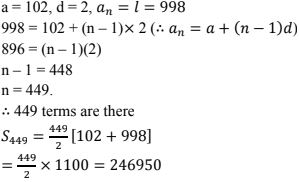∴ Sum of all even integers between 101 and  999 is 24690.

18. Find the sum of all integers between 100 and 550, which are divisible by 9.
Solution
Integers between 100 and 550 which are divisible by 9 are  108, 117, .....,549.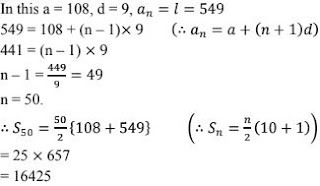∴ Sum of all integers between 100 and 550, which are divisible by 9 is 16425.

19. In an A.P., if the first term is 22, the common difference is - 4 and the sum to n terms is 64, find n.
Solution20. In an A.P., if the 5 the and 12th terms are 30 and 65 respectively, what is the sum of first 20 terms ?
Solution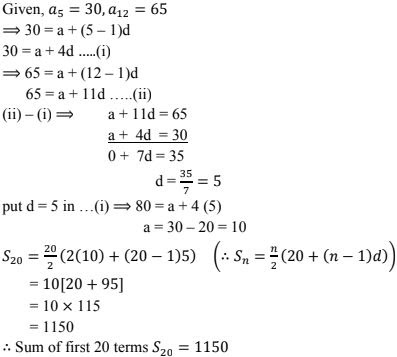21.  Find the sum of the first
(i) 11 terms of the A.P. 2, 6, 10, 14, ....
(ii) 13 terms of the A.P.  -6, 0, 6, 12, ......
(iii) 51 terms of the A.P. whose second term is  2 and fourth term is 8.
Solution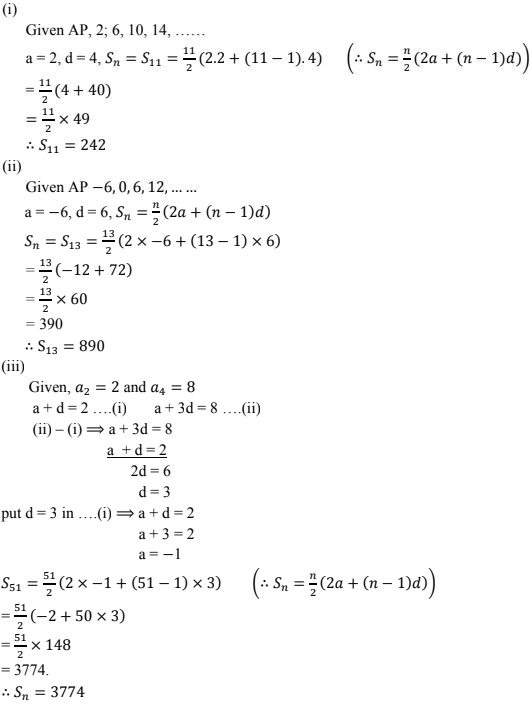22. Find the sum of
(i) the first 15 multiples of 8
(ii) the first 40 positive integers divisible by (a) 3 (b) 5 (c) 6.
(iii) all  3 digit natural numbers which are divisible by 13.
(iv) all 3 - digit natural numbers, which are multiples of 11.
Solution
The first 15 multiples of 8 are 8, 16, 24, .....
a = 8, d = 8, n = 15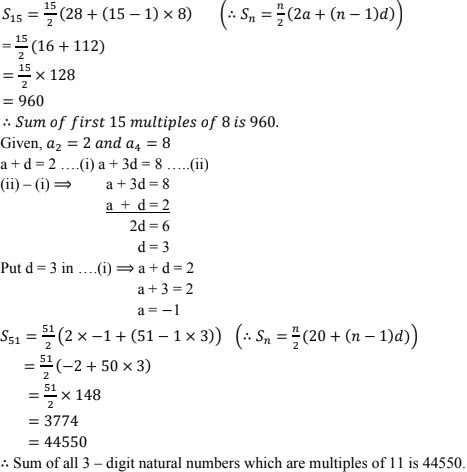23. Find the sum :
(i) 2 + 4 + 6+ ....... + 200
(ii) 3 + 11 + 19+ ...... + 803
(iii) 34 + 32 + 30 + ...... + 10
(iv) 25 + 28 + 31 + ..... + 100
Solution24. Find the sum of the first 15 terms of each of the following sequence having nth term as
(i) an = 3 + 4n
(ii) bn = 5 + 2n
(iii) Yn = 9 - 5n
Solution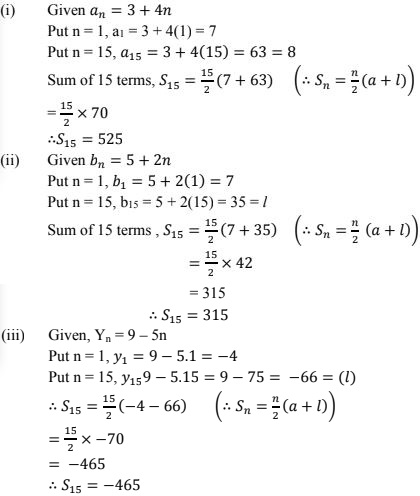25. Find the sum of first 20 terms of the sequence whose nth term is a = An + B.
Solution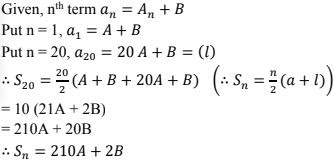26. Find the sum of the first 25 terms of an A.P. whose nth term is given by an = 2 - 3n.
Solution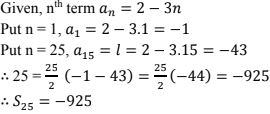27. Find the sum of the first 25 terms of an A.P. whose nth term is given by an = 7 - 3n.
Solution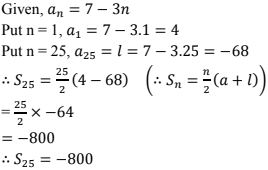28. Find the sum of first 51 terms of an A.P. whose second and third terms are 14 and 18 respectively.
Solution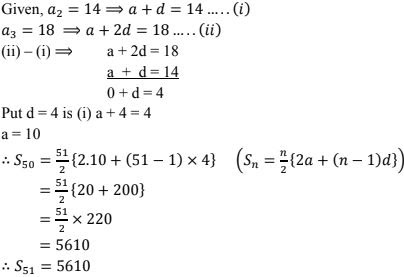29. If the sum of 7 terms of an A.P. is 49 and that of 17 terms is 289, find the sum of n terms.
Solution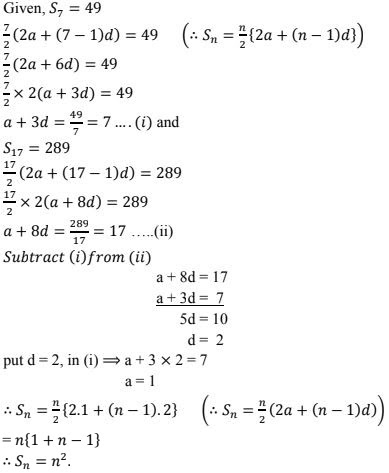30. The first term of an A.P. is 5, the last term is 45 and the sum is 400. find the number of terms and the common difference.
Solution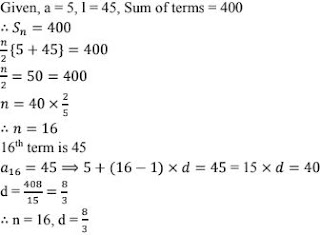31. In an A.P., the sum of first n terms is 3n2 /2 + 13n/2 . Find its 25th term.
Solution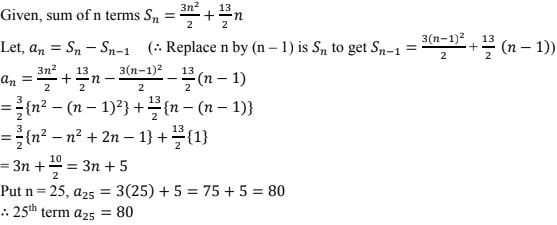32. Let there be an A.P. with first term 'a' common difference 'd'. If a denotes its nth term and sn the sum of first n terms, find.
(i) n and sn and if a = 5, d = 3 and a = 50
(ii) n and a, if an = 4, d = 2 and sn = -14
(iii) d, if a = 3, n = 8 and sn = 192
(iv) a, if an = 28, sn = 144 and n = 9
(v) n and d, if a = 8, a = 62 and sn = 210
(vi) n and an , if a = 2, d = 8 and sn = 90
Solution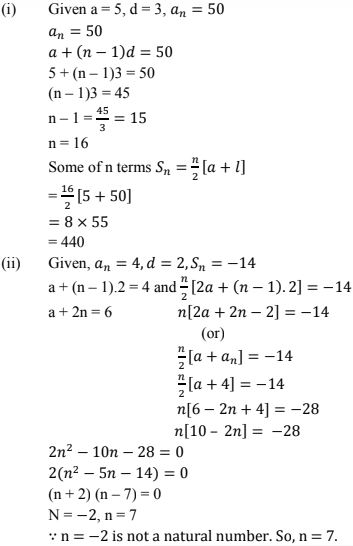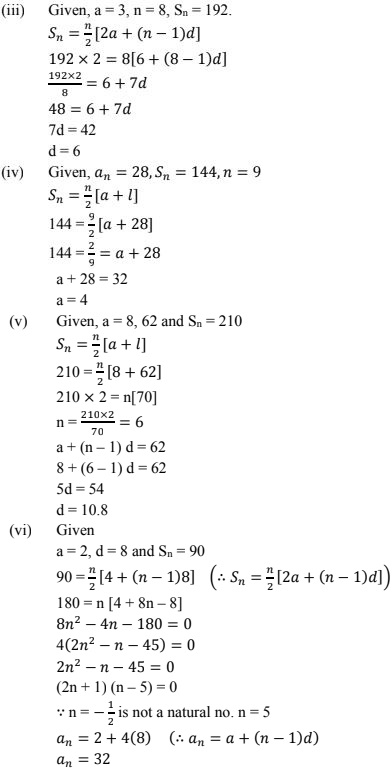33. A man saved Rs 16500 in ten years. In each years after the first he saved Rs 100 more than he did in the preceding year. How much did he save in the first year ?
Solution
Let 'a' be the money he saved in first year
⇒ First year he saved the money = Rs a
He saved Rs 100 more than, he did in preceding year.
⇒ Second year he saved the money = Rs (a + 100)
⇒ Third year he saved the money = Rs. (a + 2(100)]
So, the sequence is a, a + 100, a + 2(100), ....., This is in AP with common difference (d) = 100.
⇒ Sum of money he saved in 10 years s10 = 16500 rupees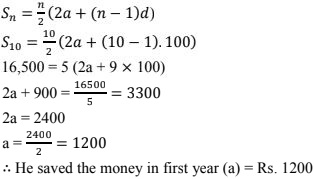34. A man saved Rs 32 during the first year, Rs 36 in the second year and in this way he increases his saving by Rs 4 every year. Find in what time his saving will be Rs 200.
Solution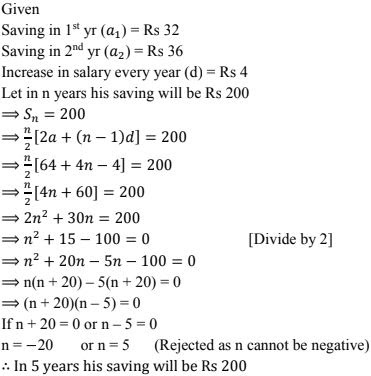35. A man arranges to pay off a debt of Rs 3600 by 40 annual installments which form an arithmetic series. When 30 of the installments are paid, he dies leaving one -third of the debt unpaid, find the value of the first installment.
Solution
Given,
A man arranges to pay off a debt of Rs 3600 by 40 annual installments which form an A.P.
i.e., sum of all 40 installments = Rs 3600
s40 = 3600
Let, the money he paid in first installment is a, and every year he paid with common difference  =d
Then,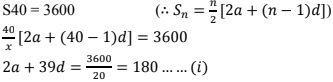but,
He died by leaving one third of the debt unpaid that means he paid remaining money in 30 installments.
∴ The money he paid in 30 installments = 3600 - 3600/3 = 3600 - 1200 = 2400
∴ s30 = 2400